### Sound and Acoustics: Sound Pressure Measurements

When sound pressure measurements are made, most commonly the actual pressure amplitudes are not measured directly. Instead, an effective pressure known as the root mean square pressure is used.

### Root Mean Square Pressure Values

The root mean square pressure (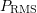) is defined as

(12.16)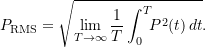This is equivalent to the square root of the average of the pressure squared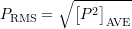which is not the same as the square root of the square of the average pressure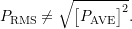For a harmonic pressure wave given by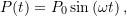we have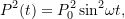so that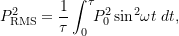whereis the associated period given by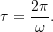Therefore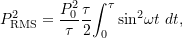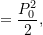so that

(12.17)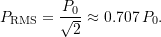These relationships are illustrated in Figure 12.2.
Note that if we have a sound composed of several harmonic waves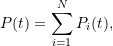where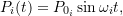then it can be shown that the RMS pressure of the resultant wave is given by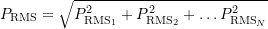where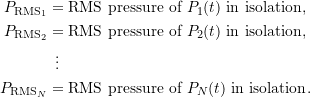Note that this only applies if the sound sources are uncorrelated.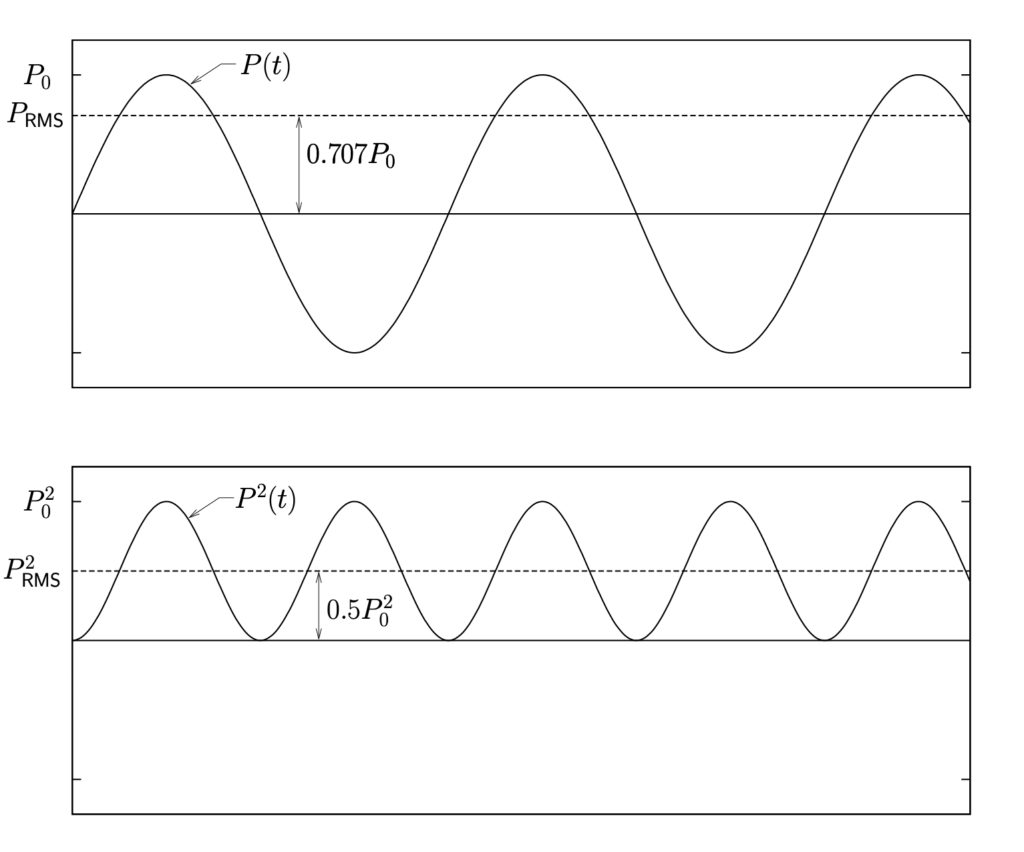Figure 12.2: Root Means Square (RMS) pressure for harmonic waves

### Superposition of Sound Waves

When two sound waves are superimposed, the result is a linear combination of the two individual waves due to the linearity of the wave equation. Two interesting situations which may occur, depending on the frequencies and amplitude of the two waves are

• Beating,
• Standing waves.

#### Beating

Beating occurs when two sounds of nearly the same frequency are combined. Consider two pressure waves with identical amplitudes and slightly different frequencies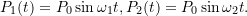When these two pressure waves are added together the total pressure is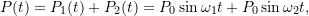or, using trigonometric identities,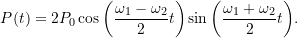By defining

(12.18)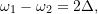this pressure response becomes

(12.19)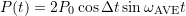where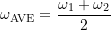is the average of the two frequencies. If the difference between the two frequencies is small,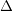will be small (much smaller than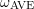) so that the response can be interpreted as a wave whose frequency is the average of the two close frequencies, but which has a slowly varying amplitude. The beating period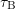is defined as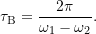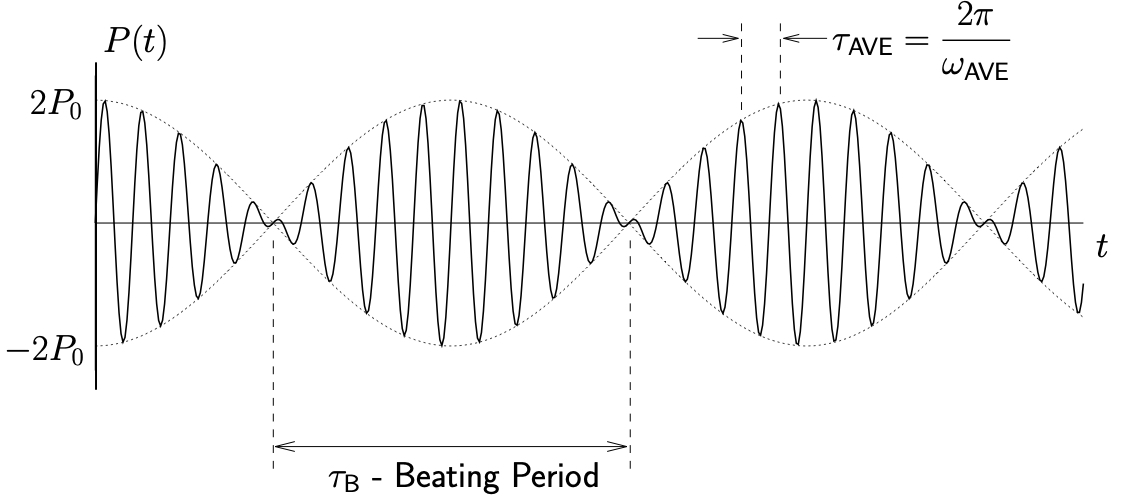Figure 12.3: Beating response for two superimposed pressure waves

A typical beating response is shown in Figure 12.3. This is similar to the beating phenomenon discussed earlier.

#### Standing Waves

Standing waves can occur when two waves travel in opposite directions. This can occur when a sound wave reflects off a surface perpendicular to the direction of the original wave propagation.
Consider two waves of equal frequency and amplitude traveling in opposite directions (These can both be shown to satisfy the wave equation 12.5.)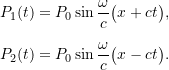The superposition of these two waves results in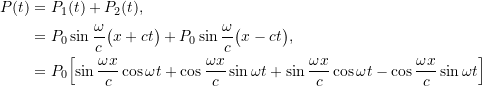or

(12.20)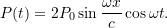However, since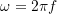and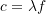where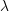is the wavelength, we see that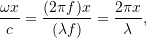so that 12.20 can be written

(12.21)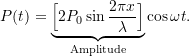Here we see that the amplitude of the pressure wave varies in space. In particular at locations given by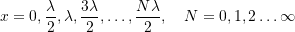we see from 12.21 that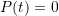so there will be no sound at these locations.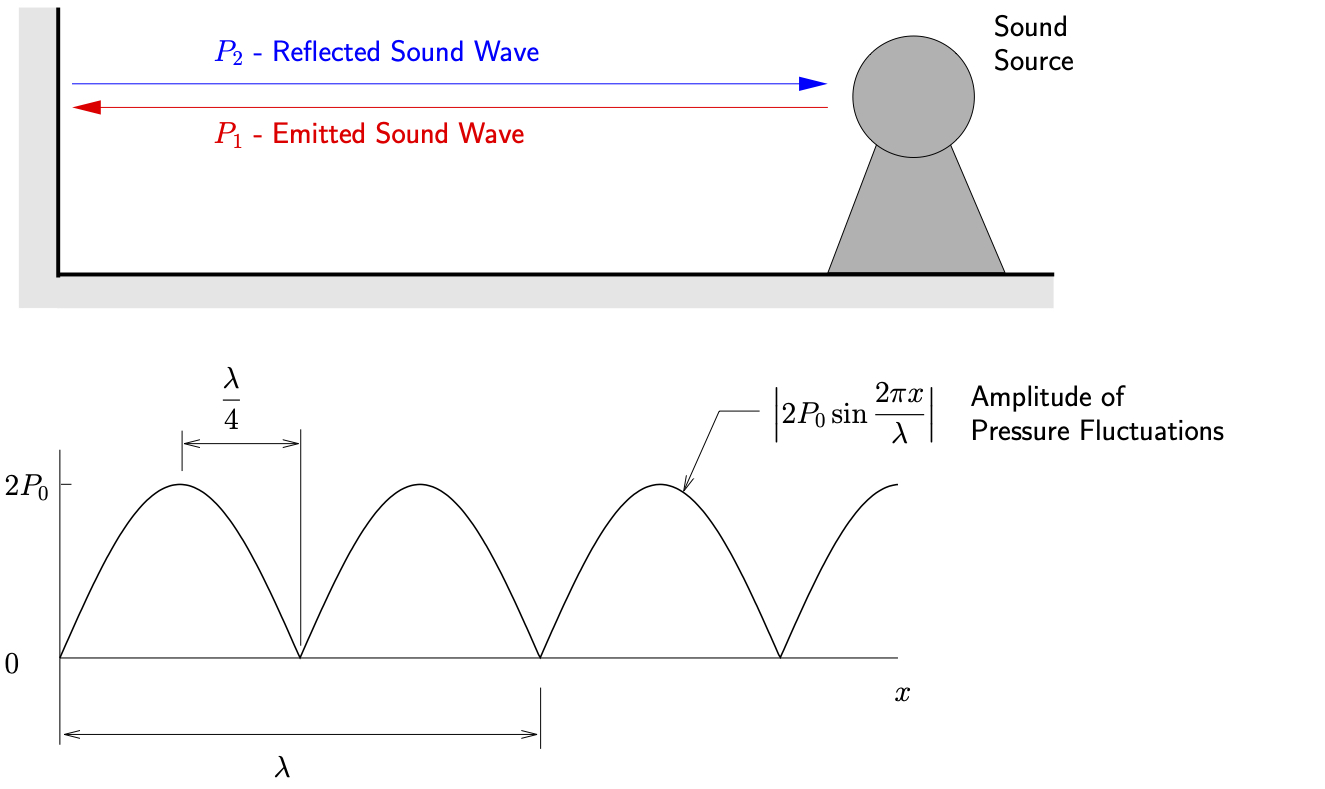Figure 12.4: Illustration of standing waves.

Consider the situation shown in Figure 12.4. As can be seen, over the distance ofof the wavelength of the sound, the pressure amplitude can change from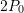to 0. To put this in perspective, for sounds at 1000 Hz for example,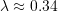\m so that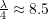\cm. This can introduce large errors in the measurement of sound pressures. These kinds of errors can occur in industrial settings where reflective surfaces are present.